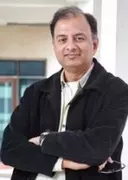X
Exact sequences in the cohomology of a Lie superalgebra extension
S. Kumar Hazra,
Published in Taylor and Francis Ltd.
2021
Abstract
Let (Formula presented.) be an abelian extension of the Lie superalgebra (Formula presented.). In this article we consider the problems of extending endomorphisms of (Formula presented.) and lifting endomorphisms of (Formula presented.) to certain endomorphisms of (Formula presented.). We connect these problems to the cohomology of (Formula presented.) with coefficients in (Formula presented.) through construction of two exact sequences which are our main results. The first exact sequence is obtained using the Hochschild–Serre spectral sequence corresponding to the above extension while to prove the second we rather take a direct approach. As an application of our results we obtain descriptions of certain automorphism groups of semidirect product Lie superalgebras. © 2021 Informa UK Limited, trading as Taylor & Francis Group.•